# How to Calculate and Solve for Magnetic Flux Density or Field Induction | The Calculator Encyclopedia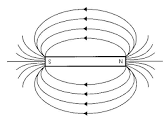The image above represents the magnetic flux density/field induction.

To compute the magnetic force of a field, four essential parameters are needed and the parameters are Magnetic Force (F), Quantity of Charge (q), Average Velocity of the Charge (v) and Angle between v and B (θ).

The formula for calculating the magnetic flux density/field induction:

B = F / qVsinθ

Where;
B = Magnetic Induction or Magnetic Flux Density
F = Magnetic Force
q = Quantity of Charge
v = Average Velocity of the charge
θ = Angle between v and B

Let’s solve an example;
Find the magnetic flux density/field induction of a field when Magnetic Force (F) is 17, Quantity of Charge (q) is 21, Average Velocity of the Charge (v) is 15 and Angle between v and B (θ) is 120°.

This implies that;
F = Magnetic Force = 17
q = Quantity of Charge = 21
v = Average Velocity of the charge = 15
θ = Angle between v and B = 120°

B = F / qVsinθ
B = 17 / (21 x 15)(sin 120°)
B = 17 / (315)(0.866)
B = 17 / (272.79)
B = 0.0623

Therefore, the magnetic flux density/field induction is 0.0623 Tesla.

Nickzom Calculator – The Calculator Encyclopedia is capable of calculating the magnetic flux density/field induction.

To get the answer and workings of the magnetic flux density/field induction using the Nickzom Calculator – The Calculator Encyclopedia. First, you need to obtain the app.

You can get this app via any of these means:

To get access to the professional version via web, you need to register and subscribe for NGN 1,500 per annum to have utter access to all functionalities.
You can also try the demo version via https://www.nickzom.org/calculator

Once, you have obtained the calculator encyclopedia app, proceed to the Calculator Map, then click on Magnetic field under the Physics section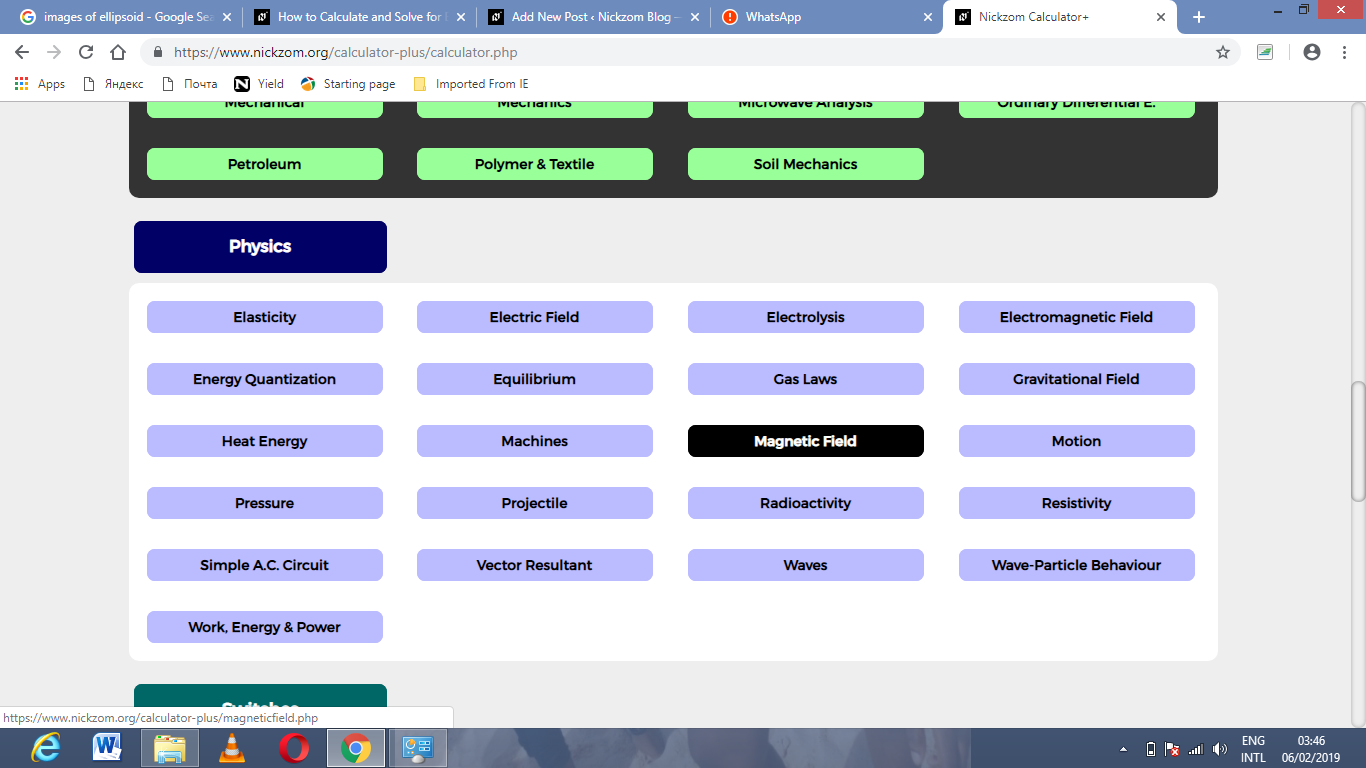Now, Click on Magnetic Flux density/field induction under Magnetic Field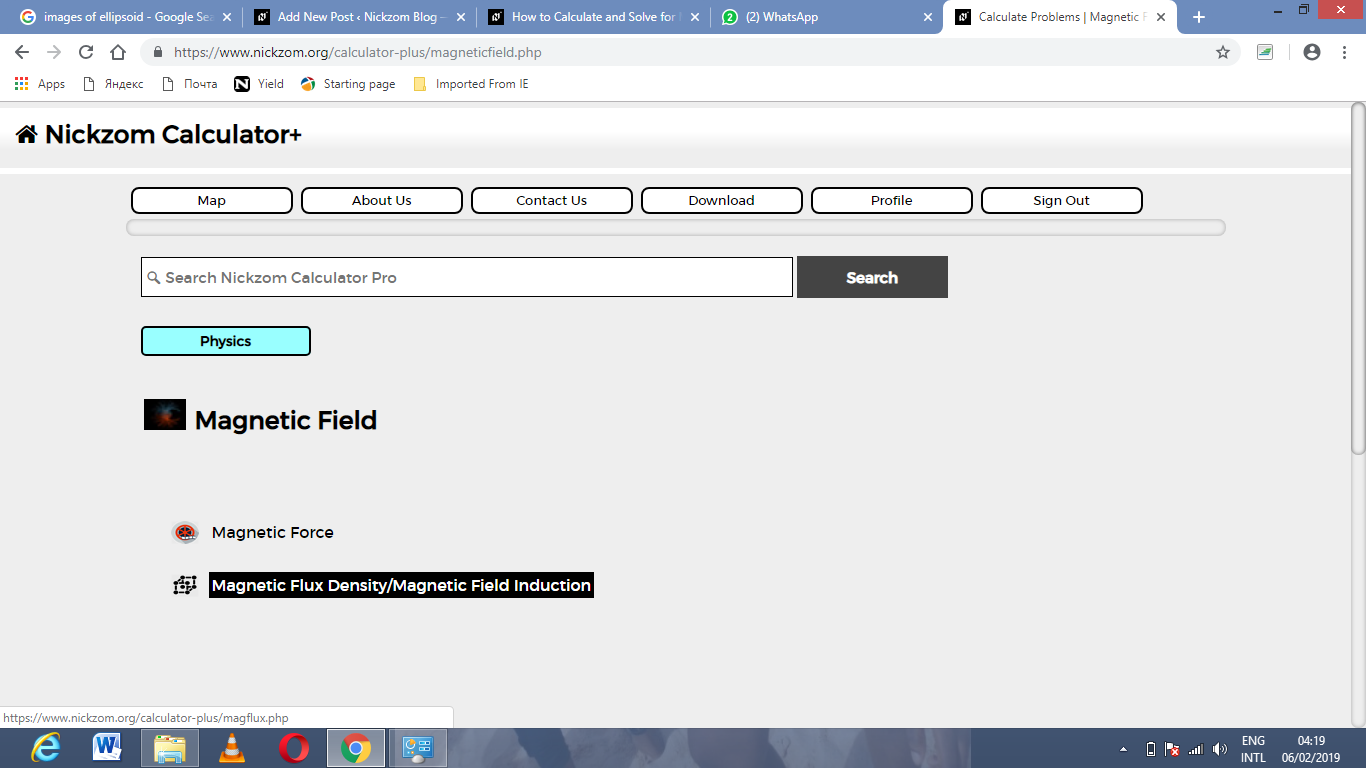The screenshot below displays the page or activity to enter your values, to get the answer for the magnetic flux density/field induction according to the respective parameters which are the Magnetic Force (F), Quantity of Charge (q), Average Velocity of the Charge (v) and Angle between v and B (θ).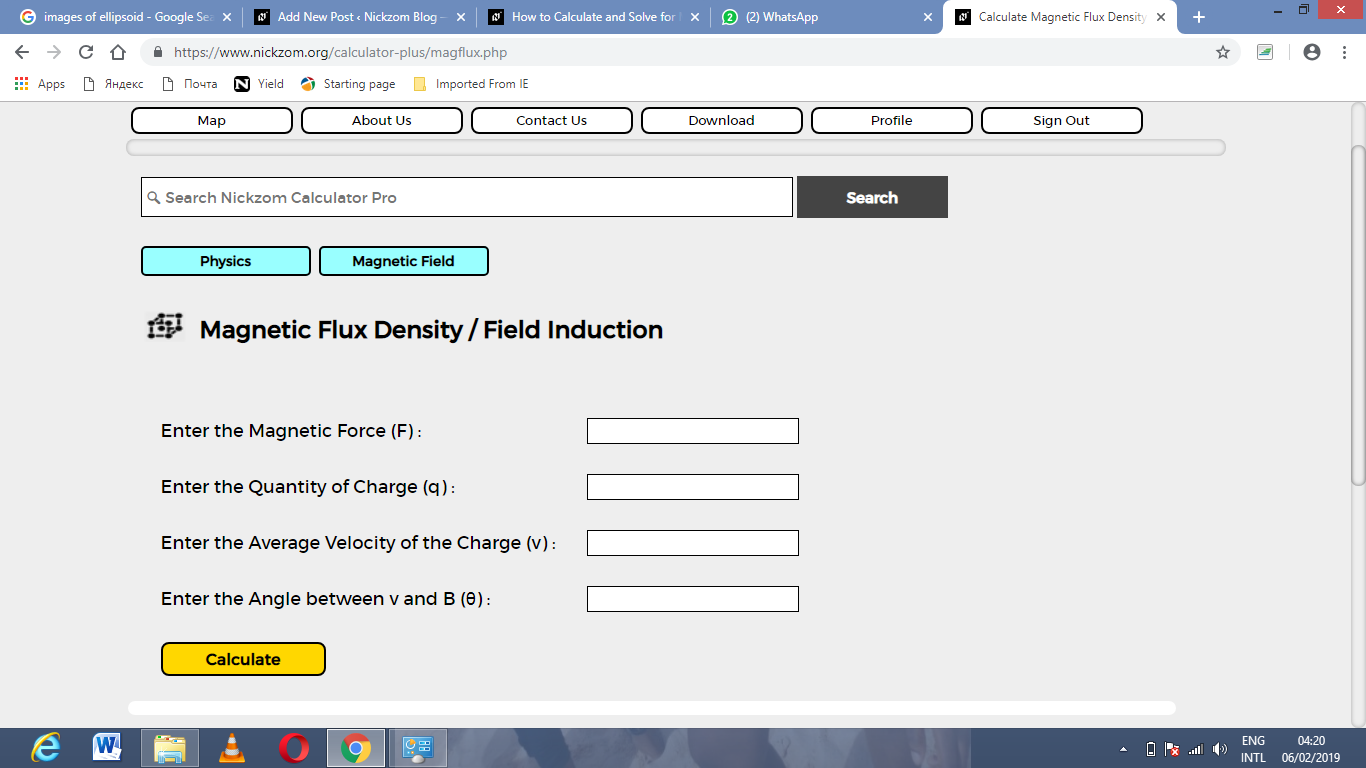Now, enter the values appropriately and accordingly for the parameters as required by the example above where the Magnetic Force (F) is 17, Quantity of Charge (q) is 21, Average Velocity of the Charge (v) is 15 and Angle between v and B (θ) is 120°.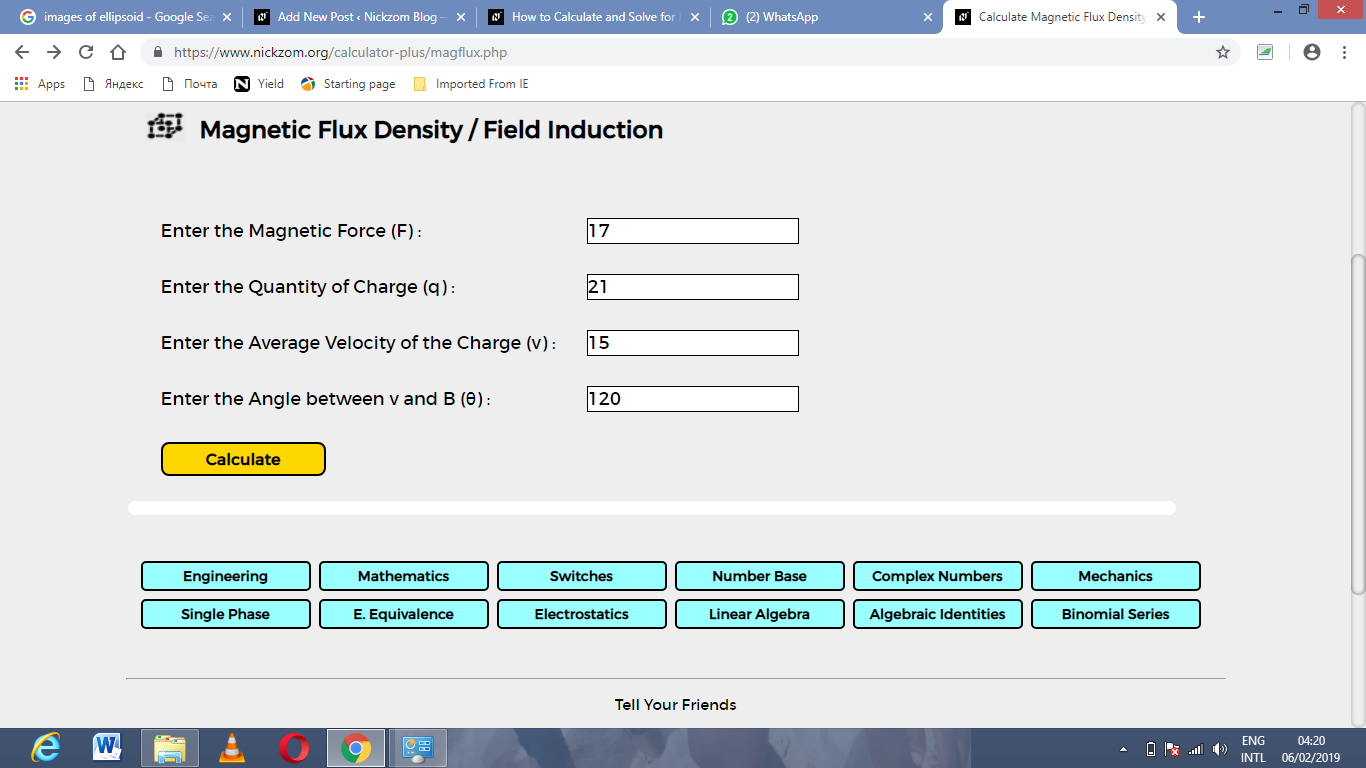Finally, Click on Calculate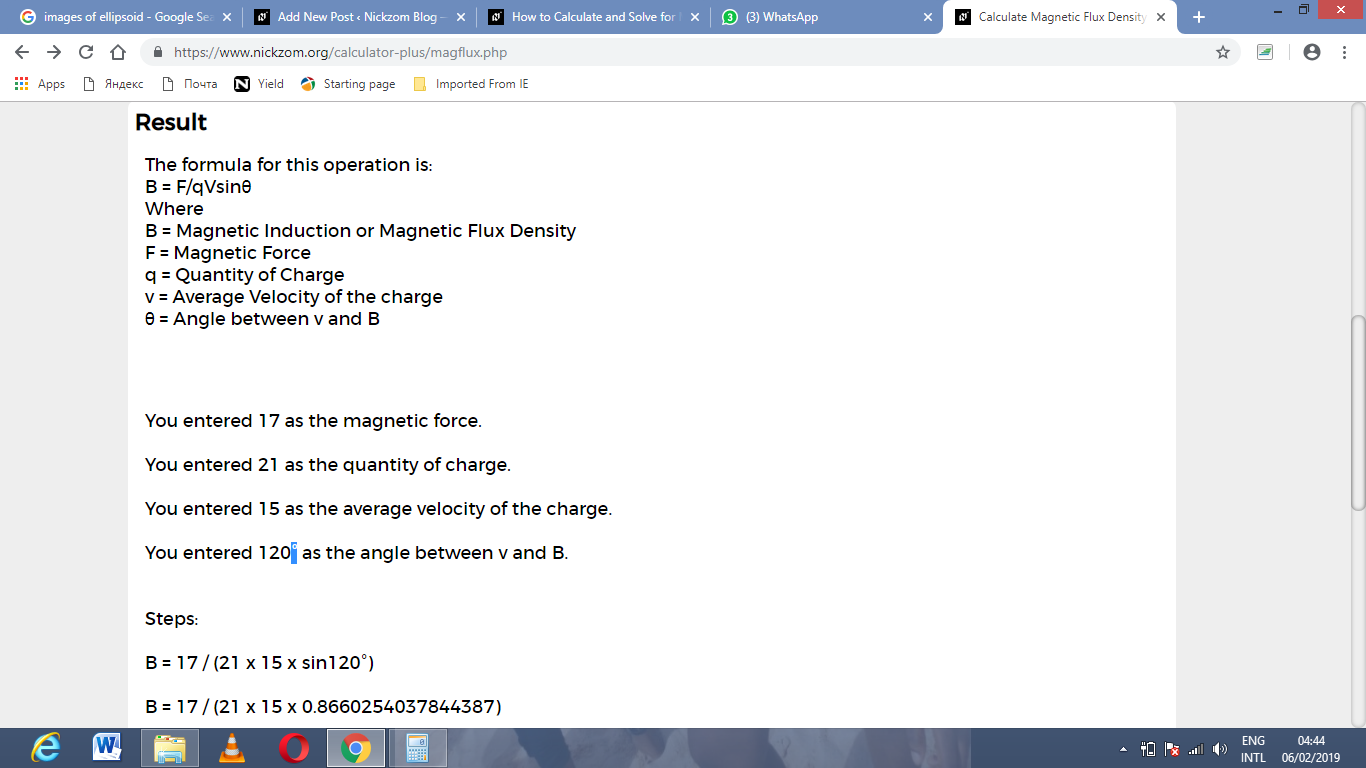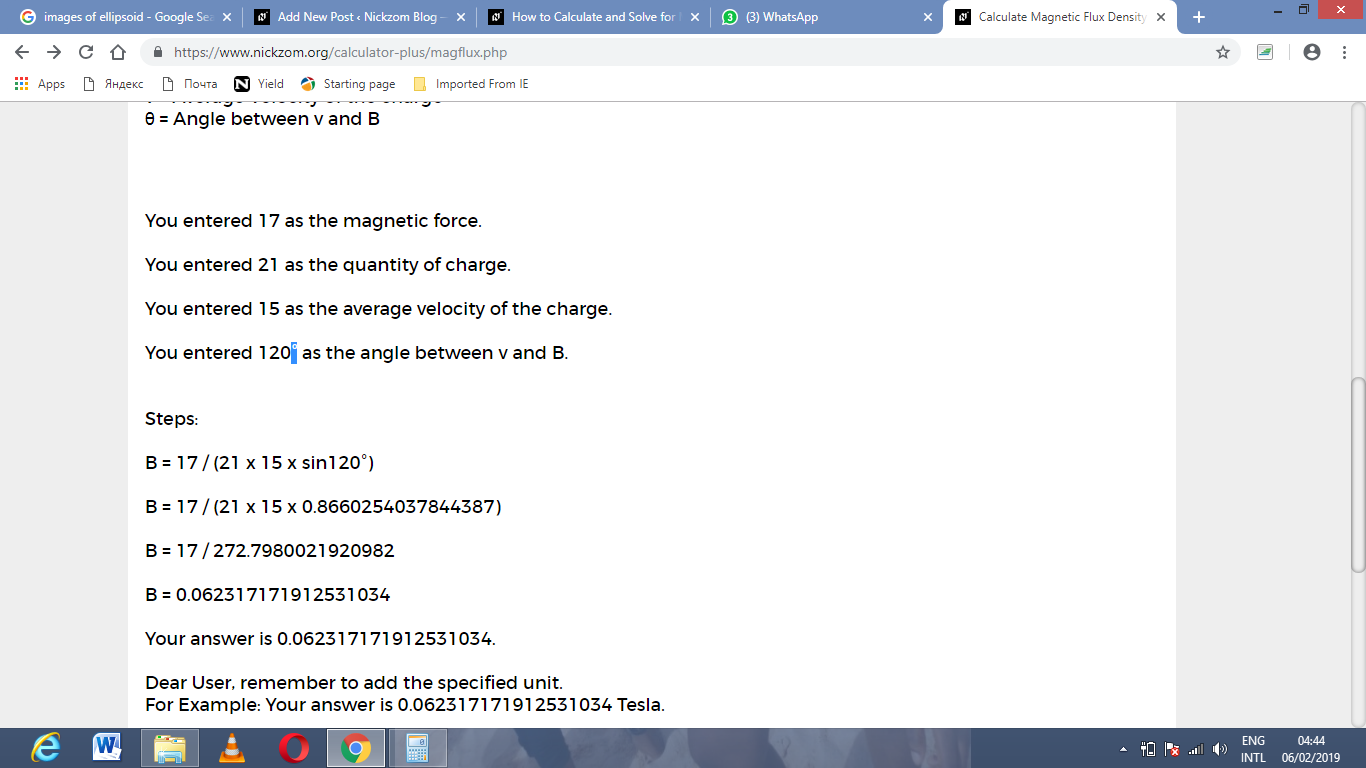As you can see from the screenshot above, Nickzom Calculator – The Calculator Encyclopedia solves for the magnetic flux density/field induction and presents the formula, workings and steps too.